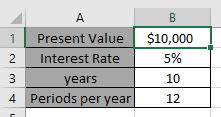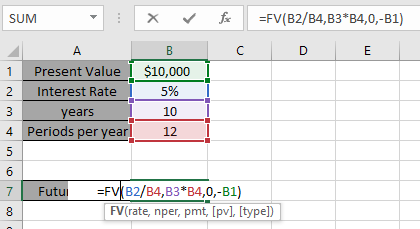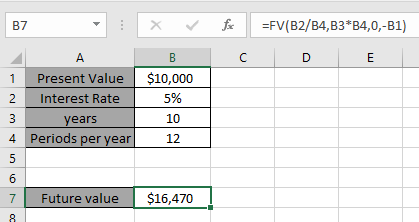# How to Use Compound Interest Function in Excel

In this article, we will learn how to use the Compound Interest function.

Compound Interest function Calculates the future value of the present amount over a period of time having an interest rate. We will be using FUTURE VALUE function here.

Future value function returns the future value of the present amount having interest rate over a period.
Syntax:

=FV (rate, nper, pmt, [pv], [type])

rate: Interest rate per period
nper: total no. of payment period.
pmt: amount paid each period.
pv - [optional] The present value of future payments must be entered as a negative number.
type - [optional] When payments are due. Default is 0.
Let’s understand this by using it in ExampleExcel Compound interest formula

=FV(B2/B4,B3*B4,0,-B1)

B2/B4: rate is divided by 12 as we are calculating interest for the monthly period.
-B1: present amount to be considered as negative to get the return is negative.Compound Interest for the following data will beAs you can see future value gets the compound interest for the data.

Hope you understood How to calculate compound interest using Future value function in Excel. Explore more articles here on Excel financial function. Please feel free to write your queries in the comment box below. We will help you.

Related Articles:

Simple interest formula in Excel

Excel IPMT Function

Calculate Profit margin percentage

Excel CUMIPMT Function

How to Use RATE Function in Excel

Popular Articles:

How to use the VLOOKUP Function in Excel

How to use the COUNTIF function in Excel 2016

How to Use SUMIF Function in Excel

Terms and Conditions of use

The applications/code on this site are distributed as is and without warranties or liability. In no event shall the owner of the copyrights, or the authors of the applications/code be liable for any loss of profit, any problems or any damage resulting from the use or evaluation of the applications/code.